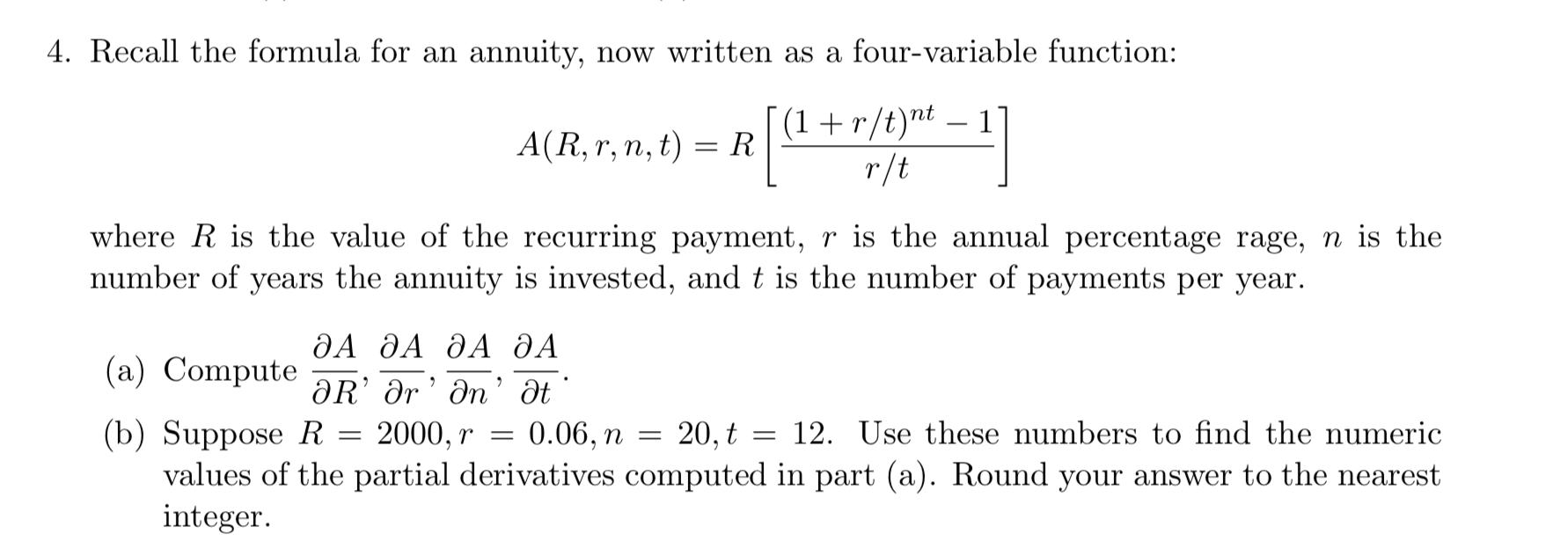# 4. Recall the formula for an annuity, now written as a four-variable function:A(R, r, n, t) = RI(1+r/tr-iwhere R is the value of the recurring payment, r is the annual percentage rage, n is thenumber of years the annuity is i ments per year., and t is the number of payДА ДА ДА ДА(a) Compute-R, Or, n. at(b) Suppose R 2000, r-0.06, п-20.ț12. Úse these numbers to find the numericvalues of the partial derivatives computed in part (a). Round your answer to the nearestinteger

Question
36 viewshelp_outlineImage Transcriptionclose4. Recall the formula for an annuity, now written as a four-variable function: A(R, r, n, t) = RI(1+r/tr-i where R is the value of the recurring payment, r is the annual percentage rage, n is the number of years the annuity is i ments per year. , and t is the number of pay ДА ДА ДА ДА (a) Compute-R, Or, n. at (b) Suppose R 2000, r-0.06, п-20.ț 12. Úse these numbers to find the numeric values of the partial derivatives computed in part (a). Round your answer to the nearest integer fullscreen
check_circle

star
star
star
star
star
1 Rating
Step 1

The formula of the annuity function with four variable R, r, n and t where, R represents the recurring payment, r represents percentage rage, n represents the number of years for which the amount invested and t represents the number of payments per year is:

Step 2

Differentiate the annuity function partially with respect to R.

Step 3

Differentiate the annuity function partially...

### Want to see the full answer?

See Solution

#### Want to see this answer and more?

Solutions are written by subject experts who are available 24/7. Questions are typically answered within 1 hour.*

See Solution
*Response times may vary by subject and question.
Tagged in

### Calculus